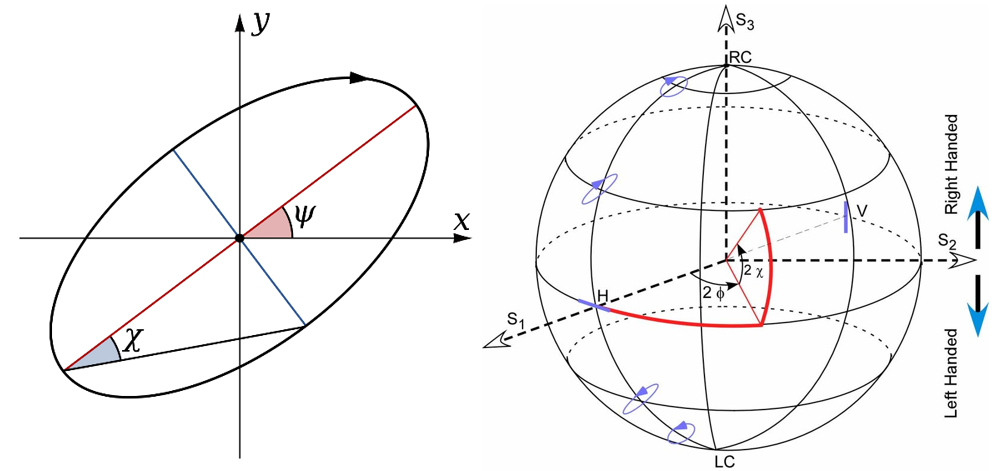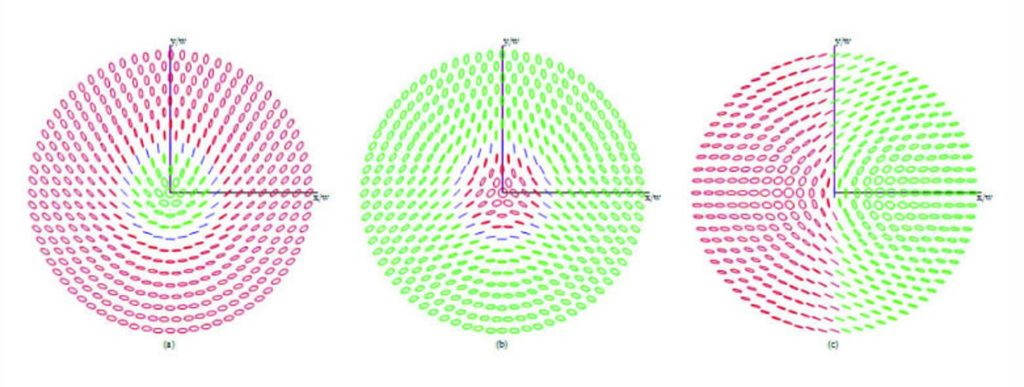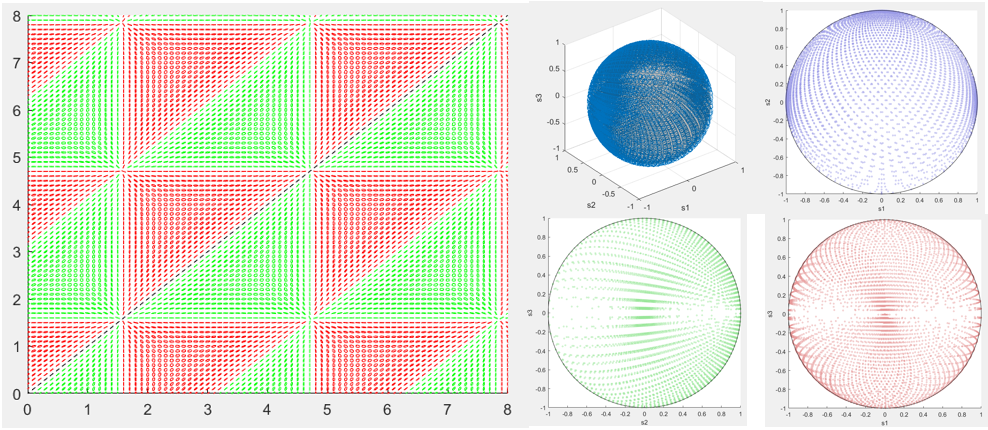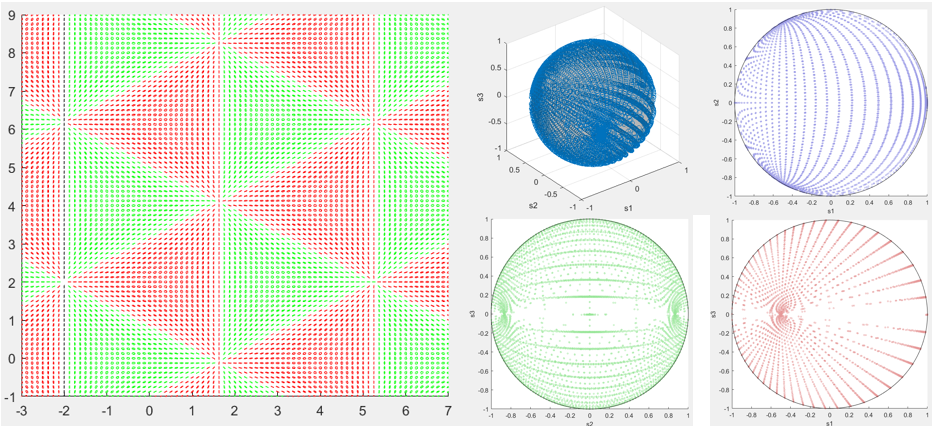Categories

# Full Poincaré Fields Composed of a Discrete Number of Plane Waves

A one or two sentence description of your project.

Lingyi Cai

Miguel Alonso

## Abstract

In recent years, full Poincaré (FP) fields have attracted an increasing amount of interest. Conventionally, the FP fields are composed of beams with Gaussian modes and Laguerre-Gaussian modes. In this thesis, we numerically and analytically analyzed the superposition of multiple paraxial plane waves and discovered the pattern of such superpositions composing FP fields. MATLAB scripts are used to simulate both the transverse plane distribution and the polarization states in the Poincaré sphere. We found that three waves are sufficient to compose a FP field, and from the FP configurations, we proposed a hypothesis of the requirements to compose FP fields with only three plane waves.

## Background

The polarization of electromagnetic waves is defined as the statistical description of the geometric behavior of the oscillations of the field vector. In isotropic media, the oscillation of the field is always perpendicular to the direction of the propagation of the wave. When multiple coherent waves are propagating in directions with a slight difference, the longitudinal polarization component will be ignorable and the polarization distribution over the transverse plane will be nonuniform.

### Poincaré sphere

In 1852, George Gabriel Stokes introduced the Stokes parameters which describe the polarization with the total intensity I, the fractional degree of polarization p, and the shape parameters of the polarization ellipse. Divide the parameters by the intensity gives the normalized Stokes vector s2D=(s1,s2,s3). When s1, s2, and s3 are used as a basis of coordinate, every polarization state represented by the normalized Stokes parameters will correspond to a single point interior of a unit sphere centered at the origin, which is called the Poincaré sphere.Figure 1.(a) Polarization ellipse showing the shape parameters. (b) Visualization of a Poincaré sphere with several polarization states labeled.

### Full Poincaré Field

Full Poincaré fields are fields with nonuniform polarization distribution which spans the entire surface when projected onto the Poincaré sphere. Amber M. Beckley, et al. (2010) first introduced that the beams can be constructed from a coaxial superposition of a fundamental Gaussian mode and a spiral-phase Laguerre-Gauss mode having orthogonal polarizations. Our goal in this thesis is to use plane waves instead to compose Full Poincaré fields.Figure 2. Evolution of the distribution of polarization ellipses for FP beams where ê_1 and ê_2 are, respectively, (a) RHC and LHC, (b) LHC and RHC, and (c) vertical and horizontal. In all movies, the radial distance is scaled by w(z) and the angle between the x-axis and the purple line represents ϕ_ξ (z).

## ResultsFigure 3. (a) Polarization distribution over the transverse plane (b) The polarization states mapped onto Poincaré sphere. (c) The plot of polarization states projected onto s1-s2 plane. (d) The plot of polarization states is projected onto s2-s3 plane. (e) The plot of polarization states projected onto s1-s3 plane.Figure 4. (a) Polarization distribution over the transverse plane (b) The polarization states mapped onto Poincaré sphere. (c) The plot of polarization states projected onto s1-s2 plane. (d) The plot of polarization states is projected onto s2-s3 plane. (e) The plot of polarization states projected onto s1-s3 plane.

## Hypothesis

Requirement for Composing FP Fields with Three Plane Waves:

For three fully polarized plane waves E1,E2,E3, exist E1, such that:

(1)k2+k3=mk1, where m∈R;

(2)e1=±(e2+e3);

(3)(k1Te2 )(k1Te3 )<0